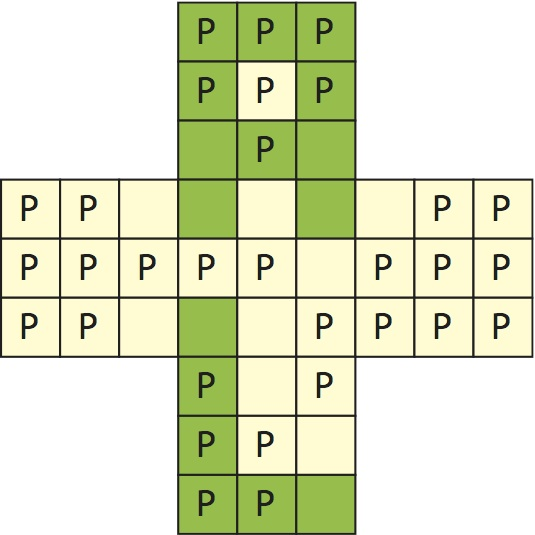Want to share your content on R-bloggers? click here if you have a blog, or here if you don't.

For the X table below, what are the minimal number of lights that are on (green) to reach the minimal and maximal possible numbers of entries (P) with an even (P as pair) number of neighbours with lights on? In the illustration below, there are 16 lights on (green) and 31 entries with an even number of on-neighbours.As suggested last week, this was amenable to a R resolution by low-tech simulated annealing although the number of configurations was not that large when accounting for symmetries. The R code is a wee bit long for full reproduction here but it works on moving away from a random filling of this cross by 0’s and 1’s, toward minimising or maximising the number of P’s, this simulated annealing loop being inserted in another loop recording the minimal number of 1’s in both cases. A first round produced 1 and 44 for the minimal and maximal numbers of P’s, respectively, associated with at least 16 and 3 1’s, respectively, but a longer run exhibited 45 for 6 1’s crossing one of the diagonals of the X, made of two aligned diagonals of the outer 3×3 tables. (This was confirmed by both Amic and Robin in Dauphine!) The next puzzle is on!